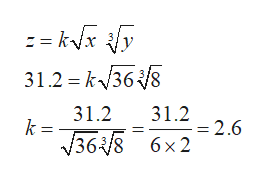# The variable z varies jointly as the square root of x and the cube root of y. If z=31.2 when x=36 and y=8, find z when x=16 and y=64

Question
41 views

The variable z varies jointly as the square root of x and the cube root of y. If z=31.2 when x=36 and y=8, find z when x=16 and y=64

check_circle

star
star
star
star
star
1 Rating
Step 1

The given joint variation can be formulated as follows.

Step 2

Given that z = 31.2 when x= 36 and y = 8. Substitute these val...help_outlineImage Transcriptionclose: = kx y 31.2 k368 31.2 31.2 =2.6 6 x2 k = 368 fullscreen

### Want to see the full answer?

See Solution

#### Want to see this answer and more?

Solutions are written by subject experts who are available 24/7. Questions are typically answered within 1 hour.*

See Solution
*Response times may vary by subject and question.
Tagged in

### Other